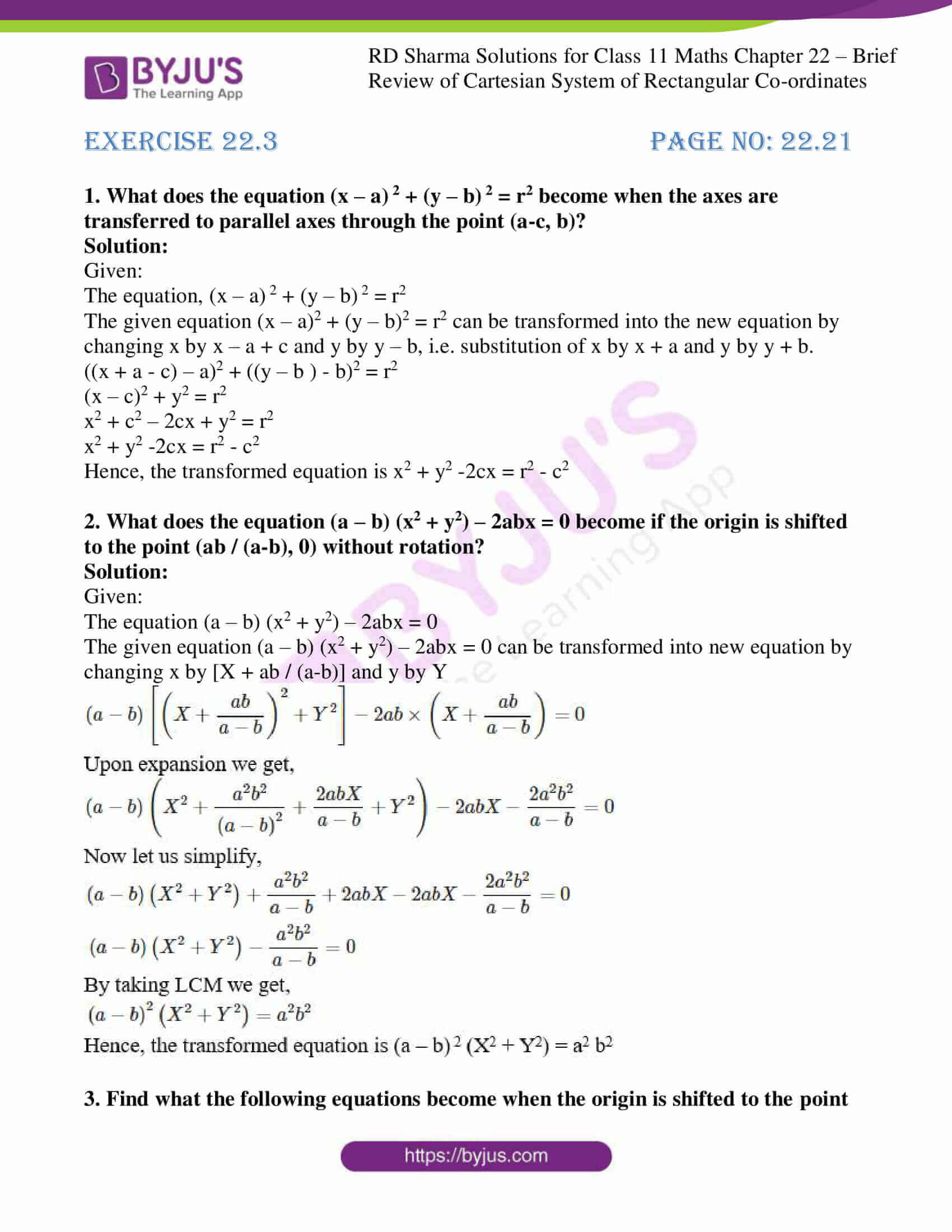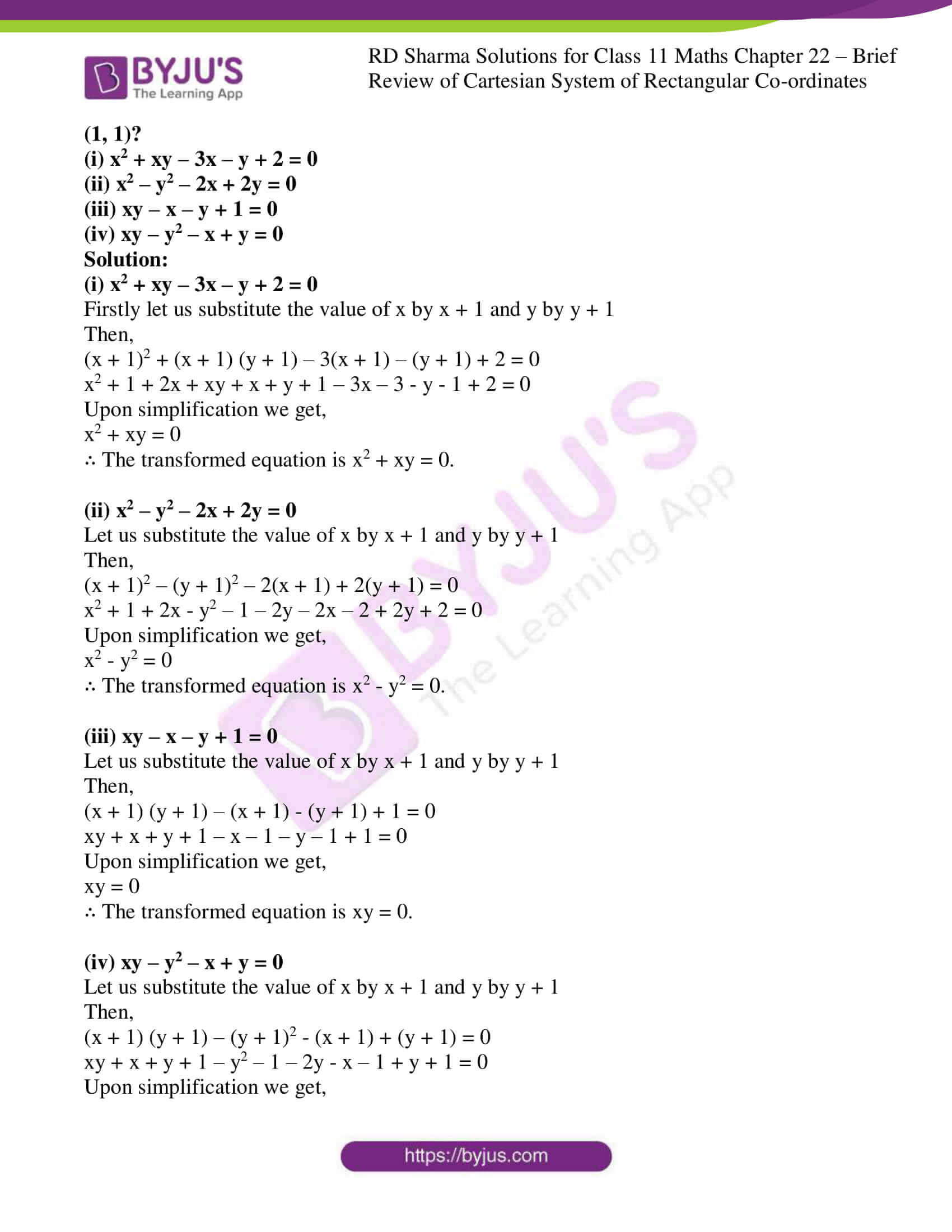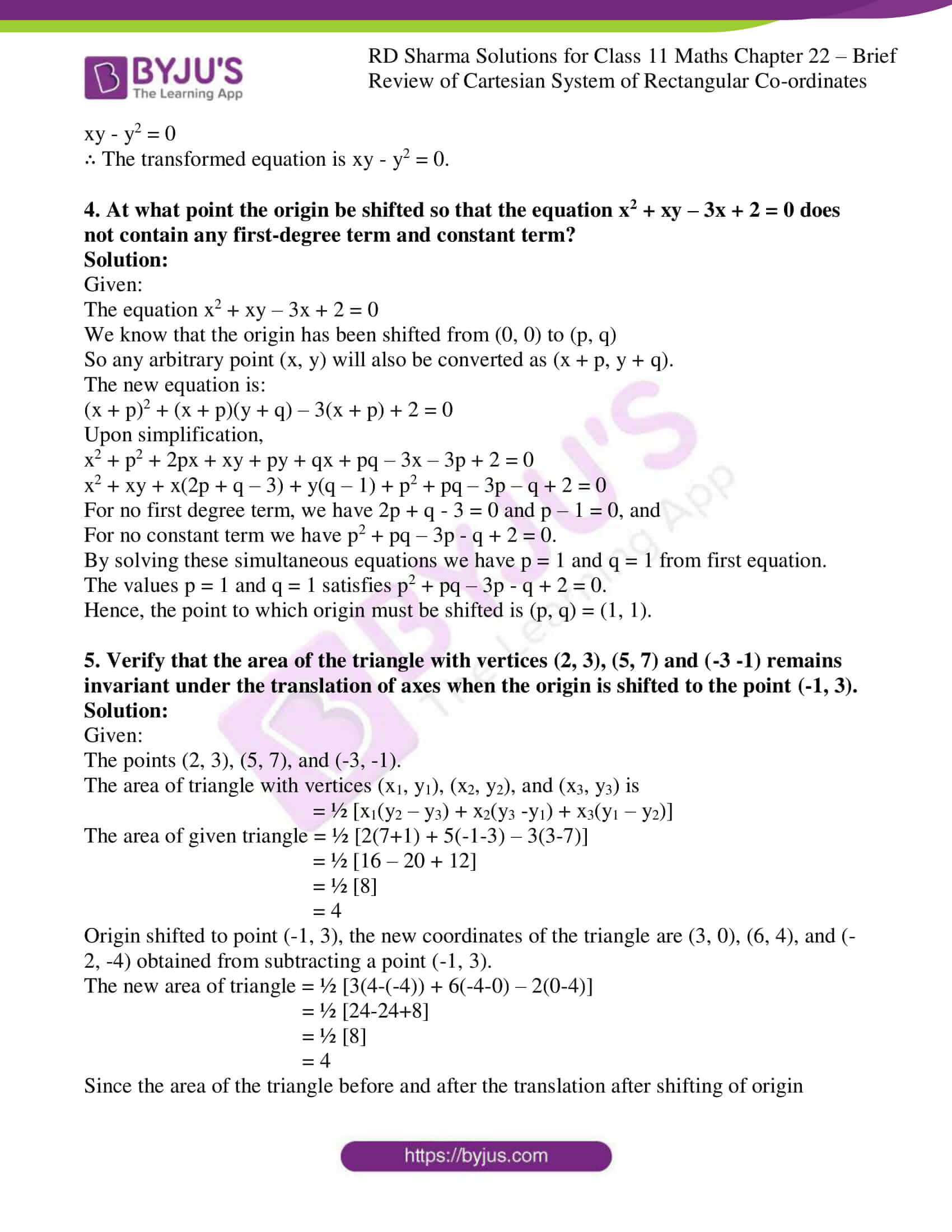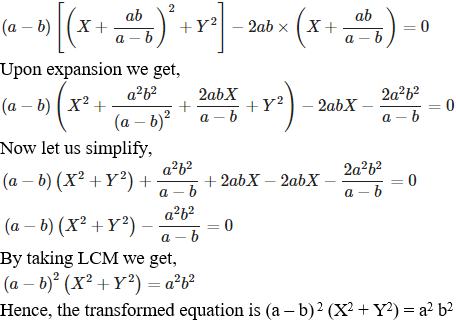# RD Sharma Solutions for Class 11 Maths Exercise 22.3 Chapter 22 - Brief review of Cartesian System of Rectangular Coordinates

RD Sharma Solutions for Class 11 Maths is an essential tool for qualifying your exams with high marks. The solutions here are designed by our team of experts at BYJU’s, in accordance with the latest CBSE norms and are explained in a simple language with suitable explanations. Those students who study at the last minute can quickly refer to these solutions, which will help in brushing up all the important concepts and the formulas. To know more about this exercise, which deals with the shifting of origin, students can download RD Sharma Class 11 Maths Solutions from the below-mentioned links.

## Download the pdf of RD Sharma Solutions for Class 11 Maths Exercise 22.3 Chapter 22 – Brief review of Cartesian System of Rectangular Coordinates### Also, access other exercises of RD Sharma Solutions for Class 11 Maths Chapter 22 – Brief review of Cartesian System of Rectangular Coordinates

Exercise 22.1 Solutions

Exercise 22.2 Solutions

### Access answers to RD Sharma Solutions for Class 11 Maths Exercise 22.3 Chapter 22 – Brief review of Cartesian System of Rectangular Coordinates

1. What does the equation (x – a) 2 + (y – b) 2 = r2 become when the axes are transferred to parallel axes through the point (a-c, b)?

Solution:

Given:

The equation, (x – a) 2 + (y – b) 2 = r2

The given equation (x – a)2 + (y – b)2 = r2 can be transformed into the new equation by changing x by x – a + c and y by y – b, i.e. substitution of x by x + a and y by y + b.

((x + a – c) – a)2 + ((y – b ) – b)2 = r2

(x – c)2 + y2 = r2

x2 + c2 – 2cx + y2 = r2

x2 + y2 -2cx = r2 – c2

Hence, the transformed equation is x2 + y2 -2cx = r2 – c2

2. What does the equation (a – b) (x2 + y2) – 2abx = 0 become if the origin is shifted to the point (ab / (a-b), 0) without rotation?

Solution:

Given:

The equation (a – b) (x2 + y2) – 2abx = 0

The given equation (a – b) (x2 + y2) – 2abx = 0 can be transformed into new equation by changing x by [X + ab / (a-b)] and y by Y3. Find what the following equations become when the origin is shifted to the point (1, 1)?
(i) x2 + xy – 3x – y + 2 = 0
(ii) x2 – y2 – 2x + 2y = 0
(iii) xy – x – y + 1 = 0
(iv) xy – y2 – x + y = 0

Solution:

(i) x2 + xy – 3x – y + 2 = 0

Firstly let us substitute the value of x by x + 1 and y by y + 1

Then,

(x + 1)2 + (x + 1) (y + 1) – 3(x + 1) – (y + 1) + 2 = 0

x2 + 1 + 2x + xy + x + y + 1 – 3x – 3 – y – 1 + 2 = 0

Upon simplification we get,

x2 + xy = 0

∴ The transformed equation is x2 + xy = 0.

(ii) x2 – y2 – 2x + 2y = 0

Let us substitute the value of x by x + 1 and y by y + 1

Then,

(x + 1)2 – (y + 1)2 – 2(x + 1) + 2(y + 1) = 0

x2 + 1 + 2x – y2 – 1 – 2y – 2x – 2 + 2y + 2 = 0

Upon simplification we get,

x2 – y2 = 0

∴ The transformed equation is x2 – y2 = 0.

(iii) xy – x – y + 1 = 0

Let us substitute the value of x by x + 1 and y by y + 1

Then,

(x + 1) (y + 1) – (x + 1) – (y + 1) + 1 = 0

xy + x + y + 1 – x – 1 – y – 1 + 1 = 0

Upon simplification we get,

xy = 0

∴ The transformed equation is xy = 0.

(iv) xy – y2 – x + y = 0

Let us substitute the value of x by x + 1 and y by y + 1

Then,

(x + 1) (y + 1) – (y + 1)2 – (x + 1) + (y + 1) = 0

xy + x + y + 1 – y2 – 1 – 2y – x – 1 + y + 1 = 0

Upon simplification we get,

xy – y2 = 0

∴ The transformed equation is xy – y2 = 0.

4. At what point the origin be shifted so that the equation x2 + xy – 3x + 2 = 0 does not contain any first-degree term and constant term?

Solution:

Given:

The equation x2 + xy – 3x + 2 = 0

We know that the origin has been shifted from (0, 0) to (p, q)

So any arbitrary point (x, y) will also be converted as (x + p, y + q).

The new equation is:

(x + p)2 + (x + p)(y + q) – 3(x + p) + 2 = 0

Upon simplification,

x2 + p2 + 2px + xy + py + qx + pq – 3x – 3p + 2 = 0

x2 + xy + x(2p + q – 3) + y(q – 1) + p2 + pq – 3p – q + 2 = 0

For no first degree term, we have 2p + q – 3 = 0 and p – 1 = 0, and

For no constant term we have p2 + pq – 3p – q + 2 = 0.

By solving these simultaneous equations we have p = 1 and q = 1 from first equation.

The values p = 1 and q = 1 satisfies p2 + pq – 3p – q + 2 = 0.

Hence, the point to which origin must be shifted is (p, q) = (1, 1).

5. Verify that the area of the triangle with vertices (2, 3), (5, 7) and (-3 -1) remains invariant under the translation of axes when the origin is shifted to the point (-1, 3).

Solution:

Given:

The points (2, 3), (5, 7), and (-3, -1).

The area of triangle with vertices (x1, y1), (x2, y2), and (x3, y3) is

= ½ [x1(y2 – y3) + x2(y3 -y1) + x3(y1 – y2)]

The area of given triangle = ½ [2(7+1) + 5(-1-3) – 3(3-7)]

= ½ [16 – 20 + 12]

= ½ 

= 4

Origin shifted to point (-1, 3), the new coordinates of the triangle are (3, 0), (6, 4), and (-2, -4) obtained from subtracting a point (-1, 3).

The new area of triangle = ½ [3(4-(-4)) + 6(-4-0) – 2(0-4)]

= ½ [24-24+8]

= ½ 

= 4

Since the area of the triangle before and after the translation after shifting of origin remains same, i.e. 4.

∴ We can say that the area of a triangle is invariant to shifting of origin.